Full Text:<3031>

Summary:<1326>

CLC number: TB302

On-line Access: 2014-05-04

Revision Accepted: 2014-03-27

Crosschecked: 2014-04-22

Cited: 4

Clicked: 5278

Citations:  Bibtex RefMan EndNote GB/T7714

 Journal of Zhejiang University SCIENCE A 2014 Vol.15 No.5 P.323-330 http://doi.org/10.1631/jzus.A1400051Measurement of thermal expansion at low temperatures using the strain gage method

 Author(s):  Ke Tang, Long Sha, Yi-jian Li, Tao Jin, Shu-juan Liu Affiliation(s):  . Institute of Refrigeration and Cryogenics, Zhejiang University, Hangzhou 310027, China Corresponding email(s):   ktang@zju.edu.cn Key Words:  Thermal expansion, Linear contraction, Strain gage method, Cryogenic temperature Share this article to： More <<< Previous Article|Next Article >>>

Ke Tang, Long Sha, Yi-jian Li, Tao Jin, Shu-juan Liu. Measurement of thermal expansion at low temperatures using the strain gage method[J]. Journal of Zhejiang University Science A, 2014, 15(5): 323-330.

@article{title="Measurement of thermal expansion at low temperatures using the strain gage method",
author="Ke Tang, Long Sha, Yi-jian Li, Tao Jin, Shu-juan Liu",
journal="Journal of Zhejiang University Science A",
volume="15",
number="5",
pages="323-330",
year="2014",
publisher="Zhejiang University Press & Springer",
doi="10.1631/jzus.A1400051"
}

%0 Journal Article
%T Measurement of thermal expansion at low temperatures using the strain gage method
%A Ke Tang
%A Long Sha
%A Yi-jian Li
%A Tao Jin
%A Shu-juan Liu
%J Journal of Zhejiang University SCIENCE A
%V 15
%N 5
%P 323-330
%@ 1673-565X
%D 2014
%I Zhejiang University Press & Springer
%DOI 10.1631/jzus.A1400051

TY - JOUR
T1 - Measurement of thermal expansion at low temperatures using the strain gage method
A1 - Ke Tang
A1 - Long Sha
A1 - Yi-jian Li
A1 - Tao Jin
A1 - Shu-juan Liu
J0 - Journal of Zhejiang University Science A
VL - 15
IS - 5
SP - 323
EP - 330
%@ 1673-565X
Y1 - 2014
PB - Zhejiang University Press & Springer
ER -
DOI - 10.1631/jzus.A1400051

Abstract:
Accurate thermal expansion data of material at low temperatures are important in material selection and structural design for a cryogenic system. In this study, an experimental setup with a proportional-integral-derivative (PID) temperature control system was developed to measure the thermal expansion of solid materials at low temperatures (77–293 K), using the strain gage method. To avoid the impact of the varied sensitivity coefficient of the strain gage with the temperature to ensure an accurate measurement, we corrected the sensitivity coefficient in the temperature range of 77–293 K, by comparing the measured thermal expansion data for 304 stainless steel with the source data from the National Institute of Standards and Technology, USA. With the corrected sensitivity coefficient of the strain gage, the measured linear contractions of oxygen-free copper become quite consistent with the NIST data (with a relative deviation of 2.37%) for the cooling-down process from 293 K to 80 K.

## 1.  Introduction

Cryogenic technology has been widely used in the aerospace industry, air separation industry, natural gas industry, and large scientific apparatus, etc. The selection of material and the structural design of cryogenic systems are very important and attract a great deal of attention. Thermal expansion is one of the key thermophysical properties of cryogenic engineering materials, since there is a significant temperature difference between its operating and manufacturing status. Accurate measurement of thermal expansion at low temperatures is necessary to ensure the security and reliability of the cryogenic systems.

The methods to measure the thermal expansion of solid material can be classified, according to an extensometer, into quartz dilatometer, interferometer, optical lever, mechanical lever, strain gages, magnetic meter, pointer meter, and capacitance micrometer methods, etc. The quartz dilatometer method is frequently used at low temperatures, because of its high accuracy (Clark, ; James et al., ; Deng and Xu, ; Tezvergil et al., ). However, it is expensive and complicated to operate, and is quite demanding on the test specimen dimensions. For a test specimen of large length, the accuracy may be affected by the potential temperature gradient along it, which then requires a cryostat to have high temperature uniformity.

The strain gage method is also commonly used to measure the thermal expansion of material at low temperatures. The thermal strain caused by temperature variation can be measured by a strain gage to reflect the linear thermal expansion features (Poore and Kesterson, ; Valentich, ; Walsh and Reed, ). Compared with the quartz dilatometer method, the strain gage method has lower requirements in the machining accuracy of the test specimen, and the impact of the non-uniform temperature field is also effectively reduced, leading to a lower operational cost. However, most existing systems control the measuring temperature by adjusting the altitude of the test specimen in the cryogenic containers (Kaufman, ), which may induce much error in temperature control and measurement. In addition, the strain gage sensitivity coefficient may vary due to low temperatures, thus the correction of the strain gage sensitivity coefficient at low temperatures must also be considered for an accurate measurement.

Based on previous studies (Poore and Kesterson, ; Valentich ; Walsh and Reed, ), an experimental setup based on the strain gage method with a proportional-integral-derivative (PID) temperature control system was developed to measure the thermal expansion of solid material at low temperatures. The temperature control system has two operating modes, i.e., stepping mode and constant rate mode. The two temperature control modes were introduced and compared, and the constant rate mode was adopted in our present measurement study. Since the variation of the sensitivity coefficient of strain gages at low temperatures is a key factor affecting the accurate measurement, it was corrected in the temperature range of 77–293 K, by comparing the measured thermal expansion data of 304 stainless steel with the source data from National Institute of Standards and Technology (NIST), USA. To verify the test apparatus and methodology, the measured linear contractions of oxygen-free copper with the corrected sensitivity coefficient of strain gages are also compared with the NIST data.

## 2.  Strain gage method

The characteristic parameters of thermal expansion mainly include the linear contraction, the mean linear coefficient of thermal expansion, and the instantaneous linear coefficient of thermal expansion. When the temperature decreases from 293 K to TT=293−T), the linear contraction is defined as the relative variation of the unit length caused by the temperature variation, i.e., ΔL/L 0, where ΔL is the length variation and L 0 is the original length at 293 K. The mean linear coefficient of thermal expansion of the test specimen is defined as the relative variation of the unit length per Kelvin from 293 K to T, expressed as α=L/L 0)(1/ΔT), and the instantaneous linear coefficient of thermal expansion is defined as the linear thermal expansion per Kelvin at T, expressed as α L =(1/L 0)(dL/dT). In a Cartesian coordinate system with the temperature as horizontal axis and the contraction as vertical axis, the slope value of the line from the initial point (293 K) to any point on the contraction-temperature curve is exactly the mean linear coefficient of thermal expansion, while the slope value of the tangent line at that point is the instantaneous linear coefficient of thermal expansion at that temperature. Since the mean linear coefficient and the instantaneous linear coefficient of thermal expansion can be achieved from the linear contraction, the discussion in the section on the test results will focus on the linear contraction.

A strain gage can measure the thermal strain on the surface of a test specimen to reflect the linear contraction of material, and the strain can be transformed into the electric signal with a high precision (Hannah and Reed, ). The strain caused by temperature variation is defined as thermal output (Scalea, ; Yasmin and Daniel, ). Without a mechanical force imposed on the test specimen, the temperature variation can lead to a length variation both in the test specimen and in the strain gage, which then induces the resistivity variation of the strain gage. The resistance variation caused by the two mechanisms can be expressed as , where R is the resistance of strain gage, ΔR is the resistance variation quantity of strain gage, K s is the sensitivity coefficient of strain gage, ϕ s is the temperature coefficient of resistance of the strain gage, and α s is the mean linear coefficient of thermal expansion of the strain gage. The thermal output can be expressed as .

Note that K s, ϕ s, and α s are all temperature-dependent variables. To eliminate the influence of ϕ s and α s due to temperature variation, two identical strain gages were mounted onto two pieces (test specimen and reference piece) in the same temperature surroundings, and then connected into a half-bridge circuit as shown in Fig. 1. U 0 is the excitation voltage, ΔU is the output voltage, R 1 is the resistance of the strain gage on the test specimen, R 2 is the resistance of the compensated strain gage on the reference piece, and R 3 and R 4 are the standard resistances.Fig.1
Schematic of the half-bridge circuit for a strain gage measurement

When the temperature varies, the thermal outputs of the two strain gages ε t1 and ε t2 are , and , where α 1 and α 2 are the mean linear coefficients of thermal expansion of the test specimen and the reference piece, respectively. According to the half-bridge principle, the acquired signal is the difference between these two thermal outputs, Δε t=ε t1ε t2=(α 1α 2T. The relationship between the mean linear coefficient of the thermal expansion of the two pieces and their thermal outputs can be written as .

Therefore, the linear contraction of the test specimen can be expressed as .

Thus, the linear contraction of the test specimen can be obtained with the measured Δε t and ΔT, as well as the mean linear coefficient of thermal expansion of the reference piece α 2. Since the fused silica glass of high purity (>99.99%) has a tiny and almost temperature-independent mean linear coefficient of thermal expansion (5.5×10−7 K−1) (Poore and Kesterson, ), it is frequently used as the reference piece in the measurement of thermal expansion.

## 3.  Experimental setup

The experimental setup to measure the thermal expansion of material at low temperatures is shown in Fig. 2, which consists of a liquid nitrogen container, a test cavity, a PID temperature regulator, a constant voltage DC source, a computer, and a data acquisition system.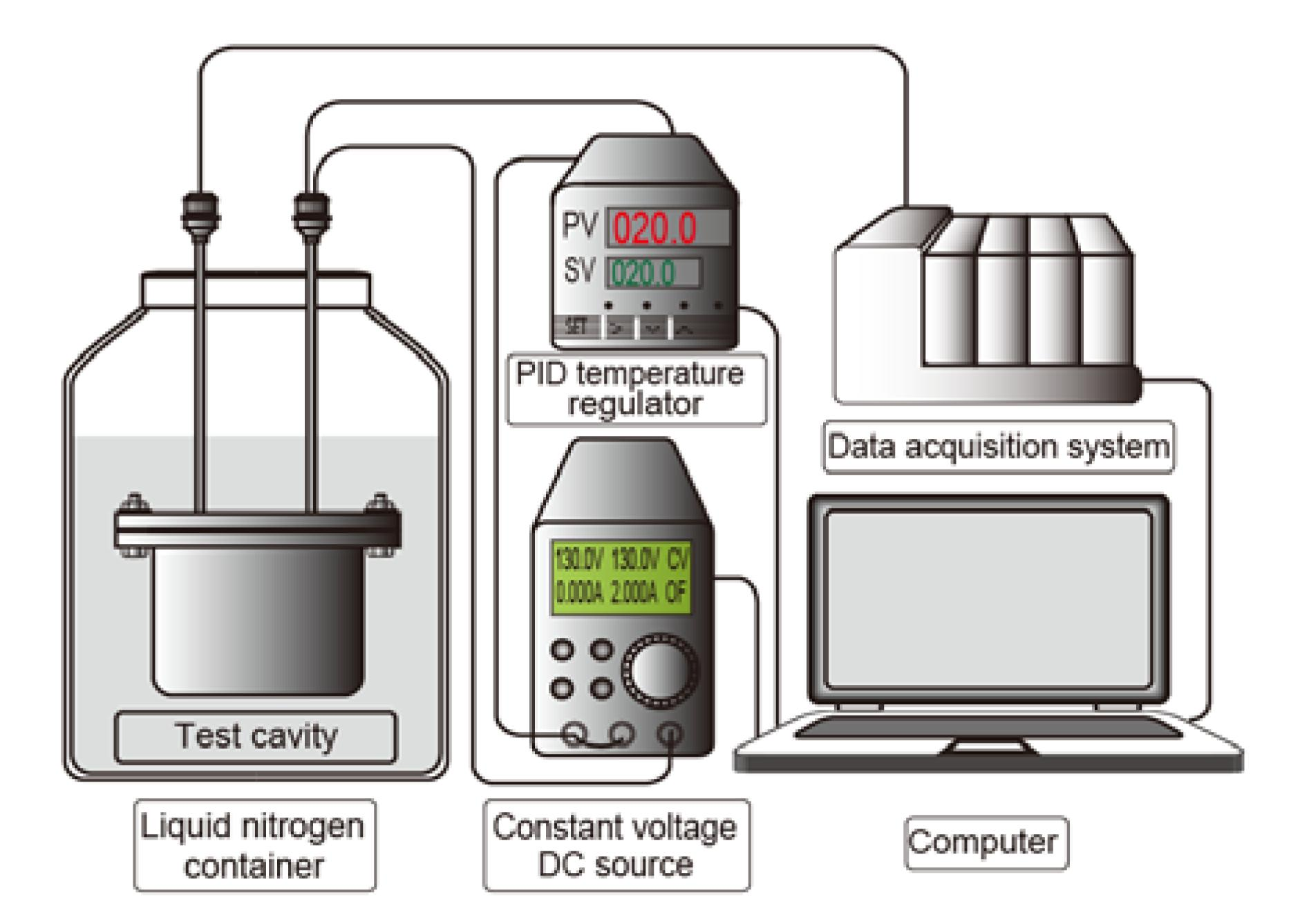Fig.2
Schematic of measurement system for thermal expansion of solid materials at low temperatures

The test cavity, as presented in Fig. 3, is mainly composed of a stainless steel chamber, thermal insulating material, a heating band, a copper cavity, a polytetrafluoroethene (PTFE) tray, a test specimen, a reference piece, and a temperature measuring block. The whole stainless steel chamber is emerged into liquid nitrogen to cool the test specimen. The heating flux supplied by the heating band can be adjusted by the PID temperature regulator to balance the heat leakage to the liquid nitrogen, resulting in a stable operation at any preset test temperature in the range of 77–293 K. The dimensions of the test specimen are 12 mm×12 mm×4 mm. Its relatively small dimensions can reduce the difficulty in machining and the influence of the non-uniform temperature field on the measurement accuracy. The reference piece with the same dimensions is made from fused silica glass of 99.99% purity. The temperature measuring block (the same material as the test specimen) also has the same dimensions, while it was drilled with holes for inserting thermometers.

Two identical strain gages were mounted at the center of the test specimen surface and the reference piece surface to measure the linear contraction. The gages were then connected into a half-bridge circuit as shown in Fig. 1. The Ni-Cr resistive elements BB120-4AA250 (11) Karma foil strain gages (Zhonghang Electronic Measuring Instruments Co. Ltd., China) were used. The design operating temperature of the strain gages is 4–523 K, the nominal resistance is (119.6±0.1) Ω, and the nominal sensitivity coefficient is 1.76±0.01. The strain signal is acquired by an NI9237 DAQ module (National Instruments, USA), which has an internal excitation source of 2.5–10 V. In addition, the NI9237 DAQ module measures the load voltage instead of the internal excitation source voltage to eliminate the error caused by wire resistance, while the shunt calibration can eliminate the error caused by the digital to analogue converter (DAC).

A PT100 platinum resistance thermometer with an accuracy of ±0.1 K, combined with an NI9217 RTD analog input module, was used for the temperature measurement. The thermometer was inserted into the temperature measuring block, which was placed at a symmetric location to the test specimen.

The test temperature control needs a cooperation of the constant voltage DC source, the heater band, the PT100 platinum resistance thermometer, the PID temperature regulator, and the computer. The PID temperature regulator and constant voltage source are connected to the computer through an RS-485 asynchronous serial communication interface. According to the standard of , the temperature can be adjusted in steps or by a slow constant rate. The temperature control system in our apparatus can achieve both operating modes. In the stepping mode, the PID temperature regulator controls the temperature by the preset P, I, and D control parameters, which can be calculated from the aforehand self-tuning experiments. Due to the large temperature range of 77–293 K, a single set of P, I, and D control parameters cannot adequately satisfy the requirements of an accurate control (a fluctuation within ±0.1 K). Therefore, several groups of parameters should be obtained by dividing the wide temperature range into relatively narrow ones. In the constant rate mode, a program based on LabVIEW software was developed to control the on/off switch timing of the relay in the temperature regulator, resulting in a relatively constant cooling-down rate for the test specimen.

## 4.  Test results and discussion

### 4.1.  Comparison of two temperature control modes

Fig. 4 presents the temperature evolution of the case controlled by the stepping mode. With the sets of P, I, and D parameters achieved from the self-tuning experiments for each 40 K temperature range, the system can be kept at diverse target temperatures with a fluctuation below ±0.1 K. A couple of hours are required to reach the stable status for each target temperature. Although the stepping mode is advantageous for accurate control of the target temperature, the long measurement time hinders its application for the measurements with variable target temperatures.

According to , the constant rate mode is available for the temperature control in the measurement of linear thermal expansion. The key point is that the temperature variation rate should be low enough to sufficiently depress the temperature’s non-uniformity, thereby ensuring the temperature measurement accuracy. The space between the stainless steel cavity and copper cavity was filled with thermal insulating material, combined with the heating band, to slow down the temperature varying rate, e.g., 0.3 K/min as shown in Fig. 5. To validate the rationality of the constant rate mode with 0.3 K/min, we compared the data for linear contractions of 304 stainless steel achieved from the constant rate mode and the stepping mode, as shown in Fig. 6. Note that there is no significant difference of the linear contractions (less than 0.26% at each target temperature) between the two modes, which indicates that the constant rate mode with 0.3 K/min is acceptable. Comparing Figs. 4 and 5, the constant rate mode takes 14 h to accomplish a relatively quick measurement. In addition, the mean rate is 0.3 K/min before reaching 83 K (12 h), and then becomes much lower because of the small temperature difference between the test specimen and the surroundings.Fig.5
Controlled temperature for constant rate mode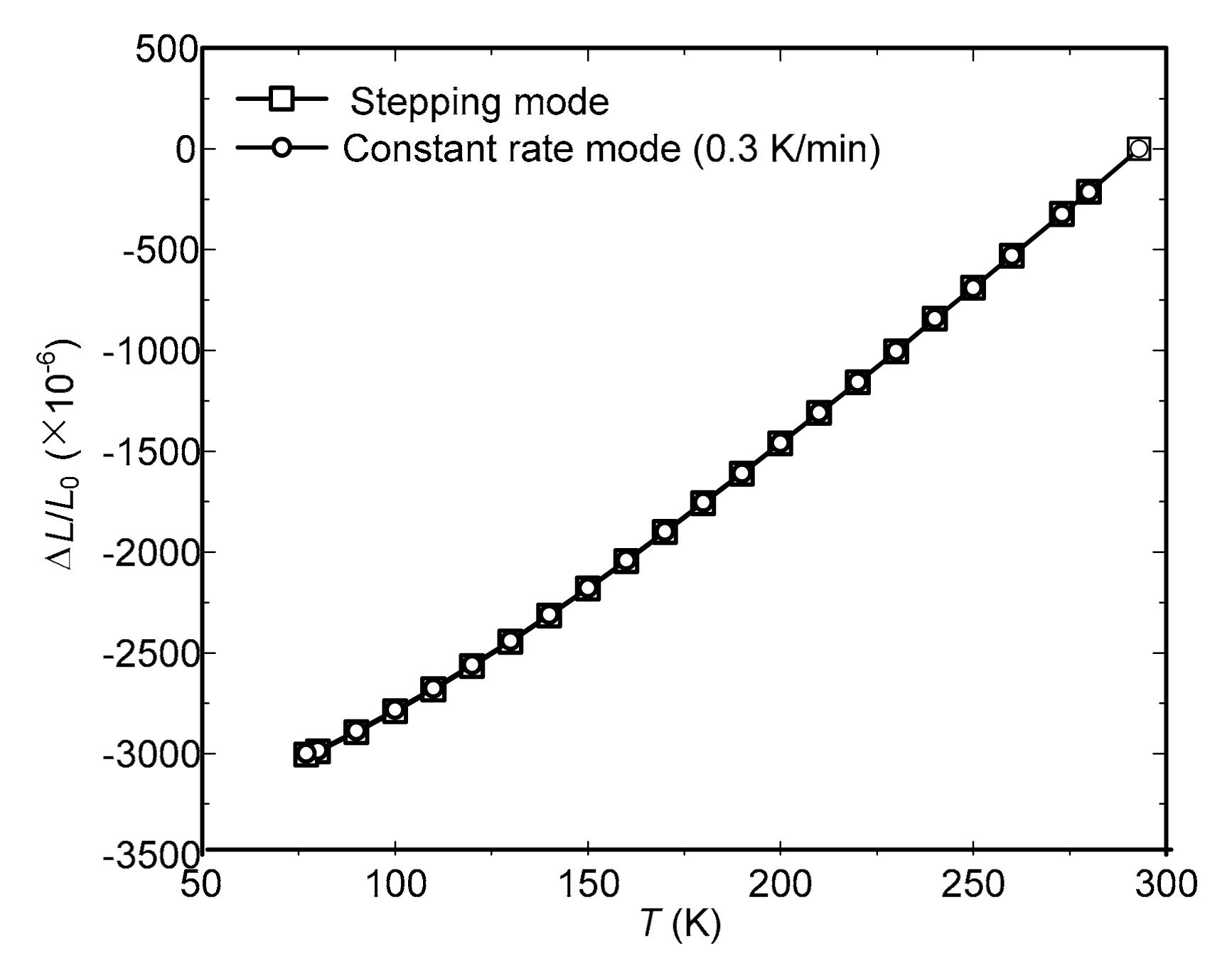Fig.6
Comparison of the two temperature control modes for the linear contractions of 304 stainless steel

Based on the above comparison, the constant rate mode with 0.3 K/min was adopted in our measurements. Assembled as in Fig. 3, the cavity was emerged into the liquid nitrogen. After the shunt calibration and zero setting for 293 K in the stepping mode, the temperature control system was then switched to the constant rate mode. Then, the variation of the linear contraction along with the temperature was acquired by the computer-based measurement and calculation application.

### 4.2.  Correction of sensitivity coefficient of strain gage for low temperatures

According to Eqs. (2)–(6), the measurement of Δε t is related to the sensitivity coefficient of strain gage K s. Since the sensitivity coefficient is significantly temperature-dependent (Yamaura, ), neglecting the variation in the sensitivity coefficient will bring errors to the measurement results for the cases of very low or very high temperatures.

To correct the sensitivity coefficient of the strain gage at low temperatures, the linear contractions of 304 stainless steel, measured in the temperature range of 293 K to 77 K with the nominal sensitivity coefficient for the room-temperature applications, were compared with the source data cited from NIST (Corruccini and Gniewek, ). As shown in Fig. 7, the deviation increases with the decrease of temperature, with a relative deviation of 6.92% at 80 K. The calculated sensitivity coefficient is 1.916 at 80 K, which has a relative deviation of 8.88% from the nominal sensitivity coefficient of 1.76. A correction coefficient defined as the ratio of the sensitivity coefficient of the strain gages at a given temperature to that at the room-temperature is presented in Fig. 8. We can find that the correction coefficient increases with the decrease of temperature, so does the corrected sensitivity coefficient.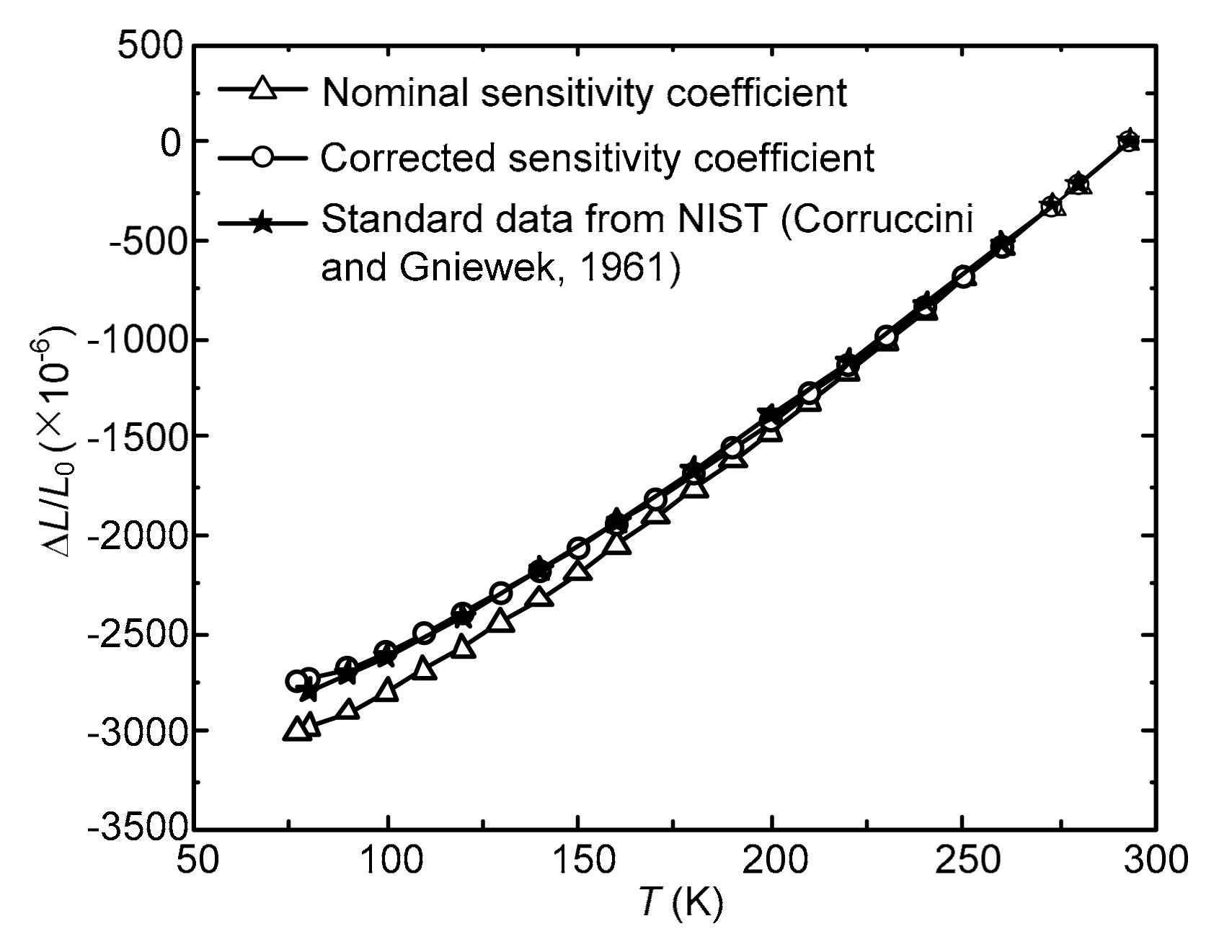Fig.7
Linear contractions relative to 293 K of 304 stainless steel in the range of 77–293 K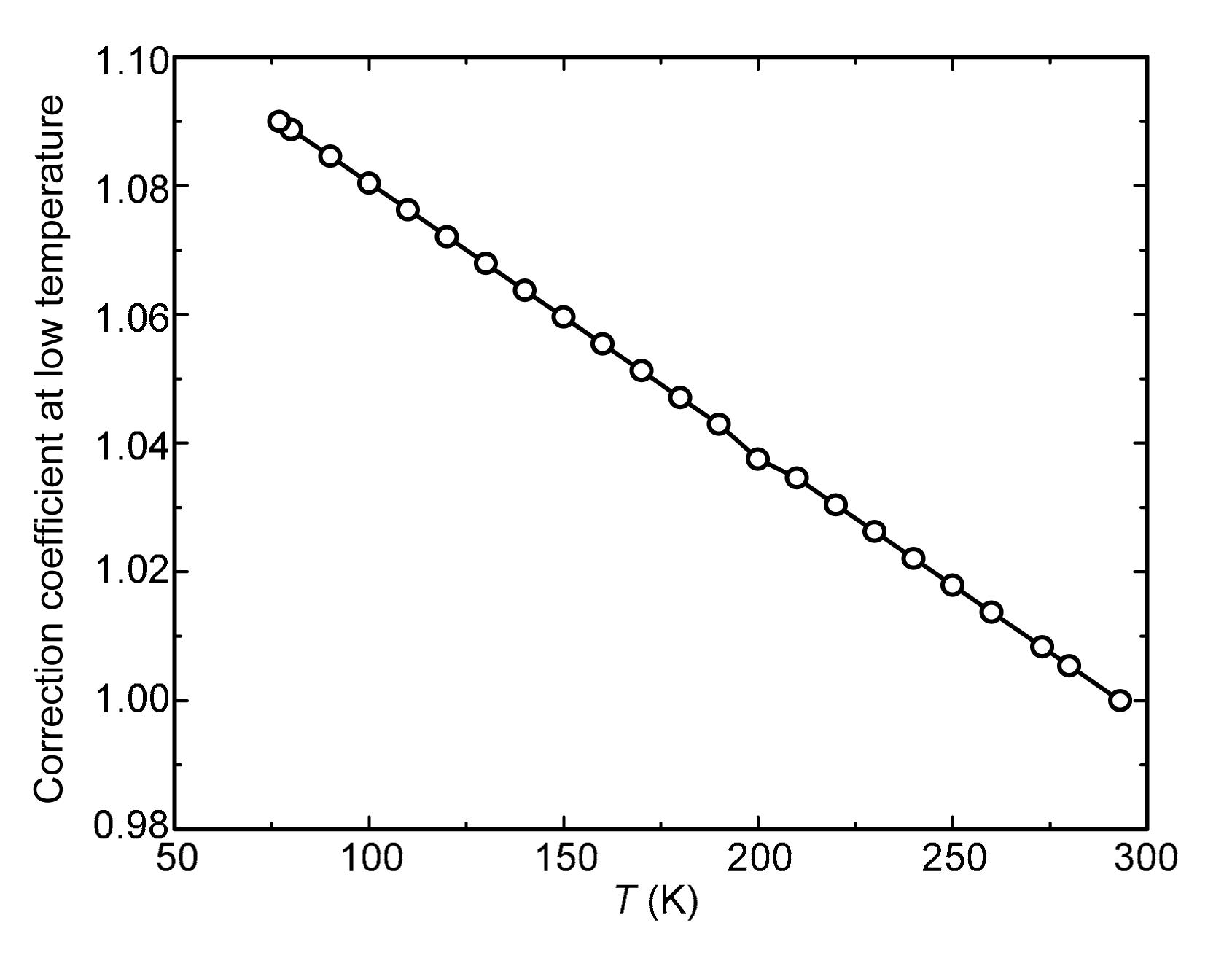Fig.8
Correction coefficient for the sensitivity coefficient at low temperatures

The linear contractions of 304 stainless steel with the corrected sensitivity coefficient are quite consistent to the source data from NIST (Corruccini and Gniewek, ), also as shown in Fig. 7. The relative deviations (absolute value) for more temperatures are given in Table 1, from which we can find that the relative deviation for the cooling-down process from 293 K to 80 K is 1.80%.

#### Table 1

Comparison of measured linear contractions relative to 293 K of 304 stainless steel in the cooling-down process from 293 K to 77 K with the source data from NIST (Corruccini and Gniewek, )
 Temperature (K) Experiment data (×10−6) NIST data (×10−6) Relative deviation (%) 293–280 −212.4 −205 3.62 293–273 −322.0 −314 2.56 293–260 −521.5 −514 1.46 293–240 −824.1 −817 0.86 293–220 −1122.4 −1110 1.11 293–200 −1405.2 −1389 1.17 293–180 −1675.5 −1670 0.33 293–160 −1933.8 −1930 0.20 293–140 −2170.9 −2180 0.42 293–120 −2387.6 −2410 0.93 293–100 −2575.8 −2610 1.31 293–90 −2661.0 −2710 1.81 293–80 −2739.8 −2790 1.80 293–77 −2750.3 293–70 −2850

### 4.3.  Validation of test apparatus

To validate the test apparatus and method for the cases at low temperatures, the linear contractions of oxygen-free copper with the corrected sensitivity coefficient in the range of 77–293 K were also measured and compared with the source data from NIST (Corruccini and Gniewek, ), as shown in Fig. 9. The relative deviations (absolute value) for more temperatures are given in Table 2, with a relative deviation of 2.37% for the cooling-down process from 293 K to 80 K, which indicates that the corrected sensitivity coefficient is applicable for diverse solid materials in the temperature range of 77–293 K.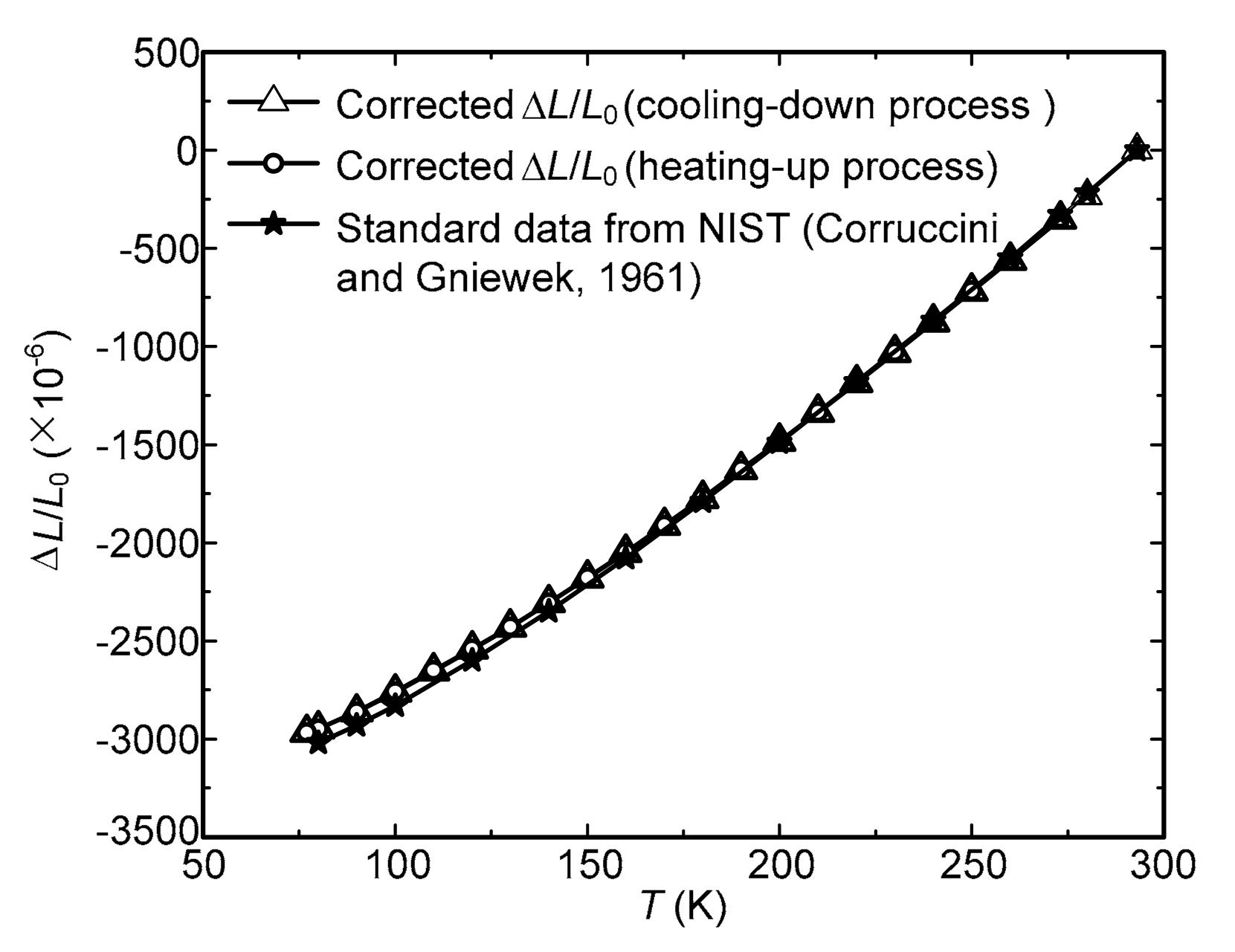Fig.9
Linear contractions relative to 293 K of oxygen-free copper in the range of 77–293 K

#### Table 2

Comparison of measured linear contractions relative to 293 K of oxygen-free copper in the cooling-down process from 293 K to 77 K with the source data from NIST (Corruccini and Gniewek, )
 Temperature (K) Experiment data (×10−6) NIST data (×10−6) Relative deviation (%) 293–280 −232.9 −220 5.85 293–273 −350.4 −330 6.18 293–260 −562.1 −550 2.20 293–240 −876.4 −870 0.73 293–220 −1185.6 −1180 0.48 293–200 −1485.3 −1490 0.32 293–180 −1775.0 −1790 0.84 293–160 −2050.9 −2080 1.40 293–140 −2370.3 −2350 1.82 293–120 −2541.7 −2600 2.24 293–100 −2762.5 −2830 2.38 293–90 −2863.4 −2930 2.27 293–80 −2948.4 −3020 2.37 293–77 −2968.2 293–70 −3100

Additionally, the measured linear contractions in the processes of cooling down and warming up are both shown in Fig. 9, which are highly consistent with a maximum deviation of 0.18%. The consistent results for the cooling-down and warming-up processes further present the indirect proof for the success of the system in the accuracy and uniformity of controlled temperatures. The rationalities of the apparatus’ thermal design and the controlled temperature varying rate (0.3 K/min) are also proven. For some alloys, the thermal expansion features are different during the processes of cooling down and warming up, due to the metallographic change. The measurement system developed in this work can be applied to measure the linear contractions during both the cooling-down and warming-up processes of those alloys.

## 5.  Conclusions

An experimental setup with a PID temperature control system based on the strain gage method has been developed to measure the thermal expansion of solid materials at low temperatures (77–293 K), with two temperature control modes, i.e., the stepping mode and constant rate mode. The relatively small dimensions of the test specimen can reduce the impact of the non-uniform temperature field on measurement accuracy. With a computer-aided measuring system, the setup is easy and at low cost to construct and operate.

For a quick and accurate measurement, the constant rate mode was adopted in our present work. Under the constant temperature varying rate of 0.3 K/min, accomplishing a relatively quick measurement requires 14 h. The sensitivity coefficient of the strain gages was corrected in the temperature range of 77–293 K, by comparing the measured thermal expansion data of 304 stainless steel with the source data from NIST. With the corrected sensitivity coefficient, the measured linear contractions of the oxygen-free copper are quite consistent to the NIST data with a relative deviation of 2.37% for the cooling-down process from 293 K to 80 K, which indicates that the corrected sensitivity coefficient is applicable for the linear contraction measurement of diverse solid materials in the temperature range of 77–293 K. Additionally, the measured data in the processes of cooling down and warming up are highly consistent with a maximum deviation of 0.18%, proving the rationality in the apparatus’ thermal design and the controlled temperature varying rate (0.3 K/min).

## ReferencesASTM E228-2006, . Standard Test Method for Linear Thermal Expansion of Solid Materials with a Push-rod Dilatometer. American Society for Testing Materials, :Clark, A.F., 1968. Low temperature thermal expansion of some metallic alloys. Cryogenics, 8(5):282-289.Corruccini, R.J., Gniewek, J.J., 1961. Thermal Expansion of Technical Solids at Low Temperatures: A Compilation from the Literature, US Department of Commerce, National Bureau of Standards,:Deng, D.Q., Xu, L., 2003. Measurements of thermal expansion coefficient of phenolic foam at low temperatures. Cryogenics, 43(8):465-468.Hannah, R.L., Reed, S.E., 1992.  Strain Gage Users Handbook. Elsevier Science Publishers Ltd.,London :James, J.D., Spittle, J.A., Brown, S.G.R., 2001. A review of measurement techniques for the thermal expansion coefficient of metals and alloys at elevated temperatures. Measurement Science and Technology, 12(3):R1Kaufman, A., 1963. Investigation of strain gages for use at cryogenic temperatures. Experimental Mechanics, 3(8):177-183.Poore, M.W., Kesterson, K.F., 1978. Measuring the thermal expansion of solids with strain gages. Journal of Testing Evaluation, 6(2):98-102.Scalea, F.L., 1998. Compensation of thermal output of strain gages on orthotropic materials: case of one compensating gage for multiple active ones. Experimental Techniques, 22(6):30-33.Tezvergil, A., Lassila, L.V.J., Vallittu, P.K., 2003. The effect of fiber orientation on the thermal expansion coefficients of fiber-reinforced composites. Dental Materials, 19(6):471-477.Valentich, J., 1985. Thermal expansion of solids from −261 °C to 173 °C using strain gauges. Cryogenics, 25(2):63-67.Walsh, R.P., Reed, R.P., 1994. Thermal expansion measurements of resins (4 K–300 K).  Advances in Cryogenic Engineering Materials. Springer,USA :1145-1151.Yamaura, Y., 1991. Measurement of strain and displacement in cryogenic environment with strain gauges. Cryogenics, (in Japanese),26(6):448-455.Yasmin, A., Daniel, I.M., 2004. Mechanical and thermal properties of graphite platelet/epoxy composites. Polymer, 45(24):8211-8219.